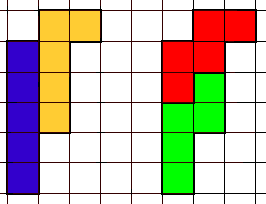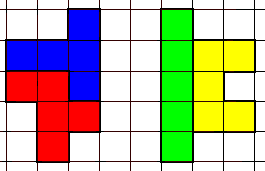Cleave Books
Pentominoes
Matching Problems

This problem requires 2 (or more) pentominoes to be put together to make one total shape, and then 2 other pentominoes found which can be put together to make the SAME total shape
In the example below, the I and L pentominoes have been fitted together on the left, and then the W and N pentominoes have been fitted together (on the right) to make the same shape.Diagrams for these two problems are given on the right.10. Find 2 pentominoes which will make the same total shape as the one given for the F and T. 11. Find 2 pentominoes which will make the same total shape as the one given for the I and U.

 12 Put V and Z together to make the same total shape as L and N. 13 Put W and X together to make the same total shape as P and Y. 14 Put V and X together to make the same total shape as U and Y. 15 Divide the full set into 3 groups. Y N L P,   F W T I,   and X U V Z. Then assemble each group into a shape so that all 3 groups show the same total shape.

 Go to PreviousPentominoProblems NextPentominoProblems PuzzlesIndex Page Cleave BooksHome Page